# Related Rates

Here's a classic related rates problem:

A ladder 10 ft long rests against a vertical wall. If the bottom of the ladder slides away from the wall at a rate of 1 ft/s, how fast is the top of te ladder sliding down the wall when the bottom of the ladder is 6 ft from the wall?

### Algebraic Solution

The standard solution is about algebra.
1. Express the relationship between the horizontal $$x$$ and vertical $$y$$ positions: $$x^2 + y^2 = 10^2$$.

2. Differentiate the expression with respect to time:
$$2x \frac{dx}{dt} + 2y \frac{dy}{dt} = 0$$. This is perhaps a bit confusing, because there are four functions involved: $$x(t)$$ and $$y(t)$$, as well as $$x^2 + y^2$$ and $$10$$.
3. Solve with respect to the desired rate: $$\frac{dy}{dt} = - \frac{x}{y}\frac{dx}{dt}$$.
4. Plug in the values for $$x$$ (which is known from the problem statement) and $$y$$ (which needs to be figured out from the relationship between $$x$$ and $$y$$) and find $$\frac{dy}{dt}$$

### Graphing the relationship

Once students know how to deal with functions of two variables, a different approach is feasible.
1. Write down the state of the system: $$x$$ and $$y$$.
2. Write down the constraint function. Or, in otherwords, write down the relationship between $$x$$ and $$y$$ and the length of the ladder: $$x^2 + y^2 = h^2$$.
3. Plot out the constraint function as a function of the state:

g = makeFun(x^2 + y^2 - h^2 ~ x + y)
plotFun(g(x, y, h = 10) ~ x + y, x.lim = c(0, 10), y.lim = c(0, 10))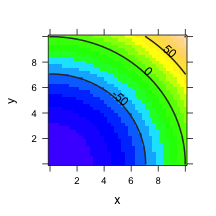1. The constraint is satisfied when the constraint function has a value of 0. Look at the zero contour on the plot.
2. Find the point on the zero contour where $$x=6$$. What's $$y$$? If you change $$x$$ from 6 to 7, what happens to $$y$$? To see this in detail, it helps to zoom in on the plot:
plotFun(g(x, y, h = 10) ~ x + y, x.lim = c(6, 7), y.lim = c(6.5,
8.5))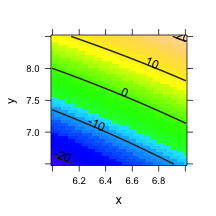You can see that a change in $$x$$ from 6 to 7 leads to a change in $$y$$ from 8 to 7.25. That the contour is approximately linear over this finite range means that the finite difference is a reasonable approximation.

The core mathematics here is about implicit functions. Given a function of $$x$$ and $$y$$ that's constant, there is implicitly a functional relationship between $$x$$ and $$y$$ (over some small domain). The length of the ladder is constant, which imposes a relationship between the ends of the ladder.

### A Textbook Example

Here's a problem from Stewart, Calculus: Concepts and Contexts 2/e p. 269

Two carts, A and B, are connected by a rope 39 feet long that pases over a pully $$P$$. The point $$Q$$ is on the floor 12 ft directly beneath $$P$$ and between the carts. Cart A is being pulled away from $$Q$$ at a speed of 2 ft/s. How fast is cart B moving toward Q at the instant when cart A is 5 feet from Q?

The state is the position of the two carts, $$A$$ and $$B$$, which we can define to be measured as the distance from $$Q$$. The constraint function is the length of the rope. Given $$A$$ and $$B$$, the rope length $$L$$ is:

rope = makeFun(sqrt(A^2 + H^2) + sqrt(B^2 + H^2) - L ~ A + B, H = 12,
L = 39)


QUESTION: How could you confirm that this is really the constraint function?

Plot out the constraint function and look for the zero contour, which is the set of values of $$A$$ and $$B$$ where the constraint is satisfied. In setting the plotting limits, you'll have to think about both the value of $$A$$ of interest ($$A=5$$) and what this implies about $$B$$. Or you can just take a guess and refine it.

plotFun(rope(A = A, B = B) ~ A + B, A.lim = c(0, 10), B.lim = c(0,
40))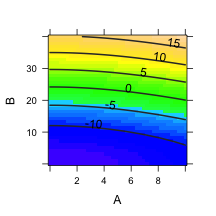QUESTIONS:
1. Let cart A move from $$A=5$$ to $$A=7$$ in one second. How far does cart B move in that time?
2. Is the relationship from $$A=5$$ to $$A=7$$ sufficiently linear that you will tolerate the approximation? Let $$A$$ change from $$A=5$$ to $$A=5.2$$ in one-tenth of a second. How far does cart B move in this time? What's the velocity of B over this second?

### Another kind of Problem

A problem from Wikipedia:
One vehicle is heading West toward an intersection at 80 miles per hour while another is heading North away from the intersection at 60 miles per hour. One can ask whether the vehicles are getting closer or further apart and at what rate at the moment when the North bound vehicle is 3 miles North of the intersection and the West bound vehicle is 4 miles East of the intersection.

The state is the positions of the two vehicles, call them $$W$$ and $$N$$. The distance between them is $$\sqrt{N^2 + W^2}$$. There is no constraint, just the distance function:

dist = makeFun(sqrt(N^2 + W^2) ~ N + W)
plotFun(dist(N = N, W = W) ~ N + W, N.lim = c(2.5, 3.5), W.lim = c(-4.5,
-3.5))
plotPoints(c(-4, -3.978) ~ c(3, 3 + 1/60), add = TRUE, pch = 20)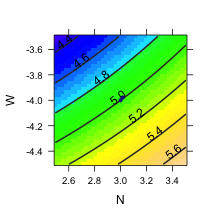The work here is in figuring out what are the relevant values of $$N$$ and $$W$$ given the statement in the word problem. Pick a relatively short time interval, say 1 seconds. The Northbound car moves 1/60 mile north during this time. The Westbound car moves $$80/3600 = 0.022$$ miles west. How much does the distance between the cars change in 10 seconds? Convert this to miles per hour.

Dots have been plotted at the position of the vehicles at the two instants. It's evident that they are at different distances: 5 miles initially to about 4.9 miles after 1 seconds. The distances can be calculated from positions:

dist(N = 3, W = -4)

##  5

dist(N = 3 + 1/60, W = -4 + 80/3600)

##  4.992


So, in 1 second, the cars move about 0.00771 miles closer, giving a closing speed of $$0.00771\times 3600 \approx 28$$ mph or about 40 feet per second.

### An Exercise

A problem from Stewart, Calculus: Concepts and Contexts 2/e p. 269:

The altitude of a triangle is increasing at a rate of 1 cm/min while the area of the triangle is increasing at a rate of 2 cm2 /min. At what rate is the base of the triangle changing when the altitude is 10 cm and the area is 100 cm2 ?

#### Approach

1. What are the known quantities? Area $$A$$, altitude $$h$$
2. What is the quantity whose rate of change is stated? $$h$$
3. What is the quantity whose rate of change it is desired to know? base $$b$$
4. What is the relationship between the quantities? $$A = \frac{1}{2}b h$$
5. Turn the relationship into a constraint function. $$A - \frac{1}{2} b h$$
6. Plot the constraint function as a function of the quantity whose rate of change is known and whose rate it is desired to know, that is, $$b$$ and $$h$$. Use the other known and fixed quantities as parameters. In this case, $$A=100$$ cm2 .
7. Using the zero contour of the constraint function, locate a point where $$h=10$$ cm and figure out the corresponding value of $$b$$.
8. Finite-difference technique. Plug in $$h=10.2$$ as the value of $$h$$ after 1/10 of a minute. Find the corresponding value of $$b$$. Multiply the change in $$b$$ by 10 to convert it to cm/sec.
9. Implicit function technique. To be written.
constraint = makeFun(A - b * h/2 ~ b + h, A = 100)
plotFun(constraint(b = b, h = h) ~ h + b, h.lim = c(9, 11), b.lim = c(19,
21))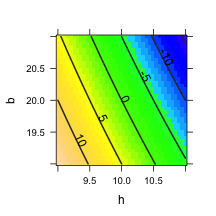### It's not always Pythagorean!

Write an example which doesn't have the Pythagorean relationship involved. For instance, imagine that the ladder is up on the side of a half-pipe. Describe the shape of the half pipe as a function parameterized in $$x$$. Let the state of the system — the end-points of the ladder — be $$x_1$$ and $$x_2$$. The constraint function is the length of the ladder as a function of $$x_1$$ and $$x_2$$.